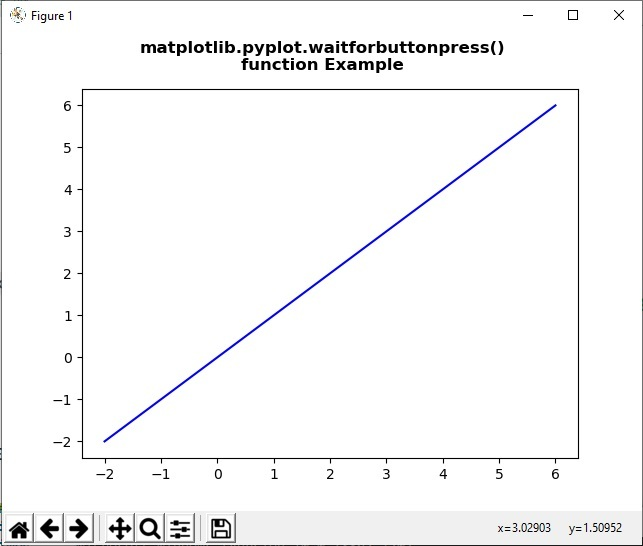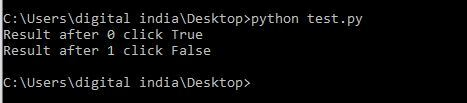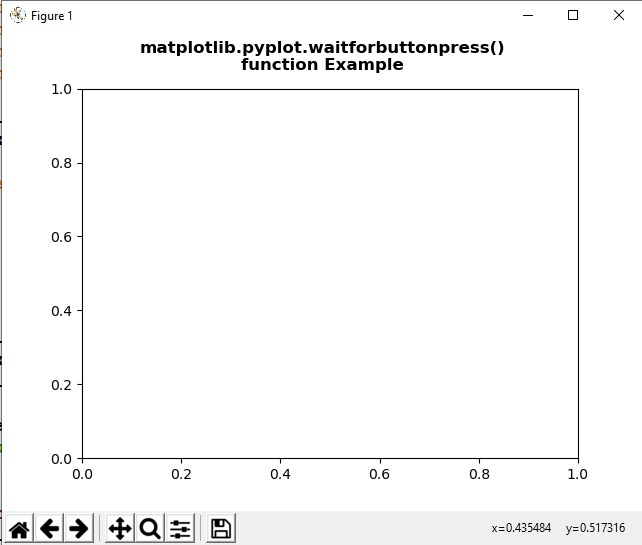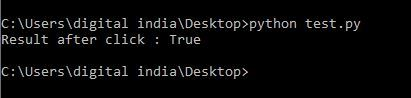# Matplotlib.pyplot.waitforbuttonpress() in Python

Matplotlib is a library in Python and it is numerical – mathematical extension for NumPy library. Pyplot is a state-based interface to a Matplotlib module which provides a MATLAB-like interface. There are various plots which can be used in Pyplot are Line Plot, Contour, Histogram, Scatter, 3D Plot, etc.

## matplotlib.pyplot.waitforbuttonpress() method

The waitforbuttonpress() method in pyplot module of matplotlib library is used for blocking call to interact with the figure.

Syntax: matplotlib.pyplot.waitforbuttonpress(timeout=- 1)

Parameters: This method accept the following parameters that are discussed below:

• timeout: This parameter is the timeout value.

Returns: This method does not returns any value.

Below examples illustrate the matplotlib.pyplot.waitforbuttonpress() function in matplotlib.pyplot:

Example 1:

 `# Implementation of matplotlib function  ` `import` `numpy as np  ` `import` `matplotlib.pyplot as plt  ` ` `  ` `  `for` `ite ``in` `range``(``2``):  ` `    ``x ``=` `np.linspace(``-``2``, ``6``, ``100``)  ` `    ``y ``=` `(ite ``+` `1``)``*``x  ` ` `  `    ``fig ``=` `plt.figure()  ` `     `  `    ``ax ``=` `fig.subplots()  ` `    ``ax.plot(x, y, ``'-b'``)  ` ` `  `    ``fig.suptitle(``"""matplotlib.pyplot.waitforbuttonpress() ` `function Example\n\n"""``, fontweight ``=``"bold")  ` ` `  `    ``w ``=` `plt.waitforbuttonpress()  ` `    ``print``(``"Result after"``, ite, ``"click"``, w)  ` ` `  `    ``plt.show()  `

Output:Example 2:

 `# Implementation of matplotlib function  ` `import` `numpy as np  ` `import` `matplotlib.cm as cm  ` `import` `matplotlib.mlab as mlab  ` `import` `matplotlib.pyplot as plt  ` `   `  ` `  `fig ``=` `plt.figure()  ` `ax ``=` `fig.subplots()  ` `   `  `def` `tellme(s):  ` `       `  `    ``fig.suptitle(s, fontweight ``=``"bold"``)  ` `    ``fig.canvas.draw()  ` `    ``renderer ``=` `fig.canvas.renderer  ` `    ``fig.draw(renderer)  ` `   `  `plt.clf()  ` `ax.axis([``-``1.``, ``1.``, ``-``1.``, ``1.``])  ` ` `  `plt.setp(plt.gca(), autoscale_on ``=` `False``)  ` `   `  `tellme(``"""matplotlib.pyplot.waitforbuttonpress() ` `function Example\n\n"""``)  ` `   `  `w ``=` `plt.waitforbuttonpress()  ` `print``(``"Result after click :"``, w)  ` ` `  `plt.show()  `

Output:Attention geek! Strengthen your foundations with the Python Programming Foundation Course and learn the basics.

To begin with, your interview preparations Enhance your Data Structures concepts with the Python DS Course.

My Personal Notes arrow_drop_upSmall things always make you to think big

If you like GeeksforGeeks and would like to contribute, you can also write an article using contribute.geeksforgeeks.org or mail your article to contribute@geeksforgeeks.org. See your article appearing on the GeeksforGeeks main page and help other Geeks.

Please Improve this article if you find anything incorrect by clicking on the "Improve Article" button below.

Article Tags :

Be the First to upvote.

Please write to us at contribute@geeksforgeeks.org to report any issue with the above content.